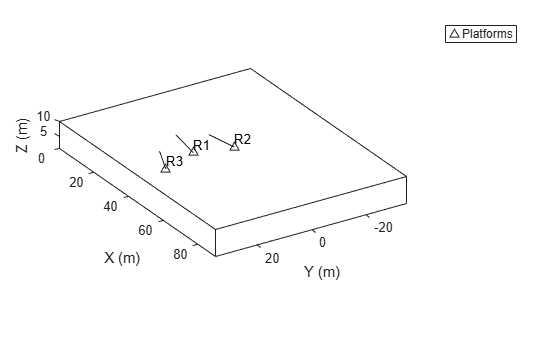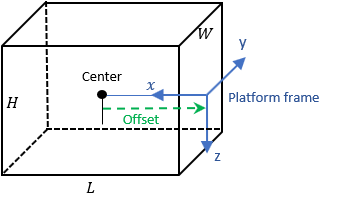# plotPlatform

Plot set of platforms in platform plotter

## Syntax

``plotPlatform(platPlotter,positions)``
``plotPlatform(platPlotter,positions,velocities)``
``plotPlatform(platPlotter,positions,labels)``
``plotPlatform(platPlotter,positions,velocities,labels)``
``plotPlatform(platPlotter,positions,___,dimensions,orientations)``
``plotPlatform(platPlotter,positions,___,meshes,orientations)``

## Description

````plotPlatform(platPlotter,positions)` specifies positions of M platforms whose positions are plotted by `platPlotter`. Specify the positions as an M-by-3 matrix, where each column of the matrix corresponds to the x-, y-, and z-coordinates of the platform locations.```
````plotPlatform(platPlotter,positions,velocities)` also specifies the corresponding velocities of the platforms. Velocities are plotted as line vectors emanating from the positions of the platforms. If specified, velocities must have the same dimensions as positions.```
````plotPlatform(platPlotter,positions,labels)` also specifies a cell vector of length M whose elements contain the text labels corresponding to the M platforms specified in the positions matrix. If omitted, no labels are plotted.```

example

````plotPlatform(platPlotter,positions,velocities,labels)` specifies velocities and text labels corresponding to the M platforms specified in the positions matrix.```
````plotPlatform(platPlotter,positions,___,dimensions,orientations)` specifies the dimension and orientation of each plotted platform.```
````plotPlatform(platPlotter,positions,___,meshes,orientations)` specifies the extent of each platform using meshes.```

## Examples

collapse all

Create a theater plot.

`tp = theaterPlot('XLim',[0,90],'YLim',[-35,35],'ZLim',[1,10]);`

Create a platform plotter with the name `'Platforms'`.

`plotter = platformPlotter(tp,'DisplayName','Platforms');`

Update the theater plot with three platforms labeled, `'R1'`, `'R2'`, and `'R3'`. Position the three platforms, in units of meters, at $\left(30,\text{\hspace{0.17em}}5,\text{\hspace{0.17em}}4\right)$, $\left(30,\text{\hspace{0.17em}}-10,\text{\hspace{0.17em}}2\right)$, and $\left(30,\text{\hspace{0.17em}}15,\text{\hspace{0.17em}}1\right)$, with corresponding velocities (in m/s) of $\left(-10,\text{\hspace{0.17em}}0,\text{\hspace{0.17em}}2\right)$, $\left(-10,\text{\hspace{0.17em}}3,\text{\hspace{0.17em}}1\right)$, and $\left(-10,\text{\hspace{0.17em}}-4,\text{\hspace{0.17em}}1\right)$, respectively.

```positions = [30, 5, 4; 30, -10, 2; 30, 15, 1]; velocities = [-10, 0, 2; -10, 3, 1; -10, -4, 1]; labels = {'R1','R2','R3'}; plotPlatform(plotter, positions, velocities, labels);```

Change the view angles to view in 3-D.

`view([-57.7 -25.9]);`## Input Arguments

collapse all

Platform plotter, specified as a `platformPlotter` object.

Platform positions, specified as an M-by-3 real-valued matrix, where M is the number of platforms. Each column of the matrix corresponds to the x-, y-, and z-coordinates of the platform locations in meters.

Platform velocities, specified as an M-by-3 real-valued matrix, where M is the number of platforms. Each column of the matrix corresponds to the x, y, and z velocities of the platforms. If specified, `velocities` must have the same dimensions as `positions`.

Platform labels, specified as an M-by-1 cell array of character vectors, where M is the number of platforms. `labels` contains the text labels corresponding to the M platforms specified in `positions`. If `labels` is omitted, no labels are plotted.

Platform dimensions, specified as an M-by-1 array of dimension structures, where M is the number of platforms. The fields of each dimension structure are:

Fields of `Dimensions`

FieldsDescription
`Length`Dimension of a cuboid along the x direction
`Width`Dimension of a cuboid along the y direction
`Height`Dimension of a cuboid along the z direction
`OriginOffset`Position of the platform coordinate frame origin with respect to the cuboid center, specified as a vector of three elementsPlatform meshes, specified as an M-element array of `extendedObjectMesh` objects.

Platform orientations, specified as a 3-by-3-by-M array of rotation matrices, or an M-element array of `quaternion` objects.

## Version History

Introduced in R2018b